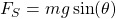# Friction Test

Tribology Wikipedia > Friction Test

In tribology, measurement of friction consists of the evaluation of forces that occur and oppose a relative motion in a tribological contact. According to ASTM standard G 40, the friction force is “the resisting force tangential to the interface between two bodies when, under the action of external force, one body moves or tends to move relative to the other”. This interface may occur between solids, solids/liquids and also may involve particles.

Since friction depends not only on the applied forces but also on the environment, each application, in general, requires a very specific friction measuring device that replicates the desired conditions. This gives rise to a large variety of devices that are built to measure friction for a specific application. All these devices can be called tribometers. Friction can be measured either directly by measuring the forces in the contact or indirectly. The list of several friction measuring configurations can be found in ASTM standard G 115 list .

The term ‘tribometre’ was introduced in the 1700s by Goldsmith. The term comes from the Greek word ‘tribos’ meaning ‘rubbing’ and “metron” meaning “measure”. Currently, tribometers can be used to quantify not only friction but also wear of the solids. The first known tribometer device can be attributed to the First Tribologist – Leonrado da Vinci, see Figure 1. His tribometer consists of pulleys and blocks. The simplest device to measure friction is probably an inclined plane with a possibility to measure the angle at which the motion of the block starts, see Figure 2. At the same time, tribometers can be as sophisticated as Atomic Force Microscopes that measures friction using piezoelectrically controlled nano-scopes tips in extremely controlled environments.

.Figure 2. Inclined plane friction measurement device

In order to perform a valid friction measurement test, one must consider the following factors:

• The measuring device should simulate the tribosystem as closely as possible
• The geometry of the test specimens should resemble the system of interest
• Conditions (contact pressure, speed, temperature, humidity, etc) of the test must resemble the ones encountered in the system of interest
• Samples should be cleaned and prepared for the test consistently
• The surface roughness of the test samples should be the same as in the evaluated tribosystem
• How to prepare samples for friction test

Friction forces often depend significantly on the surface characteristics of the tested samples. Consequently, the performance of a valid friction test requires preparation of the surfaces with characteristics representing the surfaces encountered in the evaluated tribosystem. Thus, the surface finish of the test specimens must be representative.

At the same time, the technique used to clean the surfaces prior to the test may influence the results. There is no single, best technique to prepare samples for friction testing. For instance, using solvents like acetone and ethanol for cleaning the metallic surfaces might be reasonable but similar solvents would not work well with polymers. Thus, selection needs to be made based on the material and purpose of testing.

## Friction Tests

• Inclined Plane Method

As illustrated in Figure 2, this is one of the simplest friction measurement devices that exists. This basic technique measures the inclination angle of the plane required for the relative movement of the block to start. Consequently, the static friction force equals toand the normal force equals toand therefore the static friction coefficient is given by. This coefficient is called static friction coefficient.

• Horizontal Plane Friction Test

This test often referred to as “sled test” is able to measure the friction force directly after the start of the movement (static friction force) and kinetic friction force between a slider and a flat surface during the motion. A common variation of the horizontal plane method utilizes back-and-forward movement to recreate the frictional conditions encountered in application with reciprocal motion.

• Nanofriction measurement methods

Nanometer scale friction tests have been utilized to quantify the frictional forces using a nanometer-sized probes, such as encountered in Atomic Force Microscopes. The forces rising at this scale are of importance in Microelectromechanical systems (MEMS), lithography, nanoimprinting, etc. A very thorough review of the nanofriction test measurements in particular and nanotribology in general can be found in “Tribology on the Small Scale”, by C. Mathew Mate and R. W. Carpick (2019).

• Standardized test methods

The wide range of applications where friction plays an important role has brought to the life various measurement tests standards. The standards, such as ASTM standard G 115, give the user a general understanding of the parameters that are important and precautions that need to be performed when performing friction tests. New friction standards are being created continuously with the increase in available knowledge.

Besides the standards, some standard tribometers (devices) also exist. These devices may typically perform a test on a component scale. An example of such tool would be a pin on disk test machine, which is available at various tribometer manufacturers, e.g. RTEC Instruments, Bruker, Optimol Instruments, etc.

References:

 Standard Guide for Measuring and Reporting Friction Coefficients, ASTM Standard G 115

 Tribology on the Small Scale: A Modern Textbook on Friction, Lubrication, and Wear, Second Edition, C. Mathew Mate and Robert W. Carpick, Oxford University Press, 2019.

 Blau P.J. (2013) Friction Measurement. In: Wang Q.J., Chung YW. (eds) Encyclopedia of Tribology. Springer, Boston, MA. https://doi.org/10.1007/978-0-387-92897-5_171

•
•
•
•